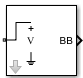# Outport

Convert RF Blockset signal to Simulink output signals

• Library:
• RF Blockset / Circuit Envelope / Utilities

•## Description

The Outport block outputs carrier modulation signals in the RF Blockset™ circuit envelope simulation environment as Simulink® signal. For an introduction to RF simulation, see the example, Simulate High Frequency Components.

The output port senses current and voltage complex envelope or real passband signals. Complex baseband signals consist of in-phase (Ik) and quadrature (Qk) components centered around each specified center frequency fk. The Sensor type parameter determines which signal the block measures, and the Output parameter defines the format of the Simulink signal. For more information, see How to Use Outport Block.

Outport block icons are dynamic and show the current format of the output signal. For more information, see Outport Block Icons.

Note

Outport block `Real passband` output does not support frame-based processing (supported by the Configuration blocks). This block errors if the Samples per frame is more than 1 in the Configuration block.

## Parameters

expand all

Type of signal measured by sensor, specified as:

• `Ideal voltage` — The block outputs the modulations of the voltage signal at the specified Carrier frequencies in the format specified by the Output parameter. This is the recommended option to sense a signal without adding a loading impedance and changing matching conditions.

• `Ideal current` — The block outputs the modulations of the current signal at the specified Carrier frequencies in the format specified by the Output parameter.

• `Power` — The block outputs the modulations of the voltage signal at the specified Carrier frequencies and scaled with respect to the specified load impedance. This is the recommended option to sense a signal generated in RF Blockset 50 Ohm environment or a different reference impedance. When you use the power option , the output port automatically inserts a load impedance in your circuit.

`$\frac{\sqrt{\text{Re}\left({Z}_{l}\right)}}{{Z}_{l}}v\left(t\right)$`

where Zl is the value of the Load impedance (ohms) parameter.

Load impedance of RF circuit used to measure signal power, specified as a vector of positive integers in ohms. When you use the power option , the output port automatically inserts a load impedance in your circuit. When you use multiple Outport blocks as power sources at the same node in a given circuit, the resulting load is the parallel combination of the specified load impedances.

#### Dependencies

To enable this parameter, select `Power` in Sensor type.

Format of output signals, specified as one of the following:

• `Complex Baseband` — The block outputs a vector of complex-valued signals Ik(t) + j · Qk(t) at the port labeled SL. The kth element of the vector is the kth frequency specified by the Carrier frequencies parameter.

• `In-phase and Quadrature Baseband` — The block outputs two vectors of real-valued signals Ik(t) and Qk(t) at the I port and Q port, respectively. The signal at the I port contains the in-phase components, and the signal at the Q port contains the quadrature components. The kth element of the vector is the kth frequency specified by the Carrier frequencies parameter. The quadrature component of a signal with carrier frequency equal to 0 Hz is zero.

• `Magnitude and Angle Baseband` — The block outputs two real-valued vectors, whose elements are the magnitude and phase angle of the modulation. The Mag port outputs |Ik(t) + j · Qk(t)| and the Ang port outputs Arg[Ik(t) + j · Qk(t)]. The kth element of the vector is the kth frequency specified by the Carrier frequencies parameter.

• `Real Passband` — The block outputs real passband signals by combining envelope and carrier signals for all frequencies listed under Carrier frequencies. When using the `Real Passband` option, the solver takes time steps small enough to resolve the carrier. Thus, simulation speed improvements from envelope simulation may be limited.

`$out=\mathrm{Re}al\left(\sum _{k=1}^{N}\left({I}_{k}\left(t\right)+j·{Q}_{k}\left(t\right)\right)·{e}^{j2\pi {f}_{k}t}\right)$`

where `t` is the value of Load impedance (ohms) parameter.

Determine optimal time step to resolve highest listed carrier frequency, specified as a `on` or `off`. Select this parameter to allow RF Blockset to determine the optimal time step to resolve the highest listed carrier frequency. Clear the parameter selection to enter a value for step size.

Time step, specified as a positive integer in seconds. The step size should be small enough to resolve the fastest carrier signal. The size helps to avoid passband output undersampling and aliasing effects.

Set the time step value to `-1` to inherit the time step specified from `Step size` in Configuration block.

Carrier frequencies, specified as a vector of positive integers in Hz. In carrier frequencies, the elements are a combination of fundamental tones and corresponding harmonics in the Configuration block.

Ground RF circuit terminals, specified as a `on` or `off`. Select this parameter to ground and hide the negative terminals. Clear the parameter to expose the negative terminals. By exposing these terminals, you can connect them to other parts of your model.

By default, this option is selected.

expand all

expand all

## Version History

Introduced in R2010b

expand all